Publicité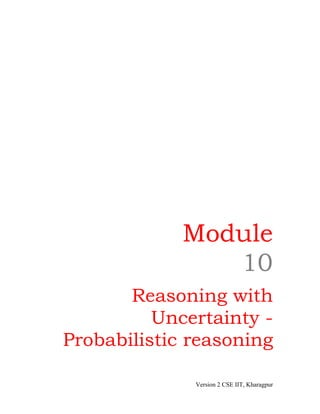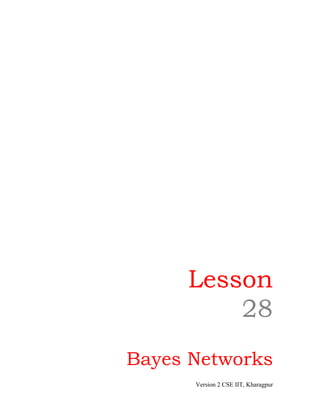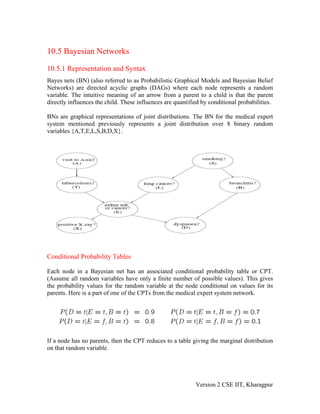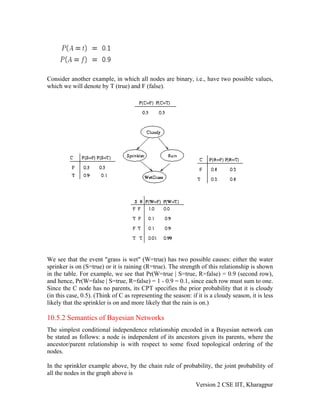Publicité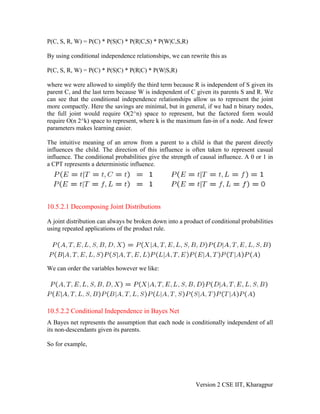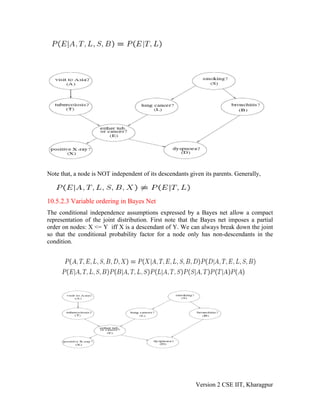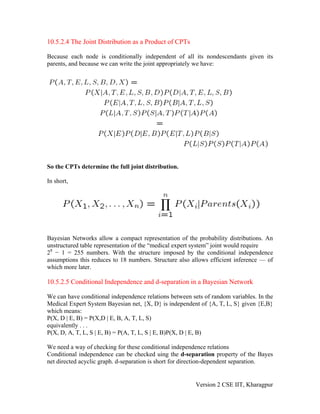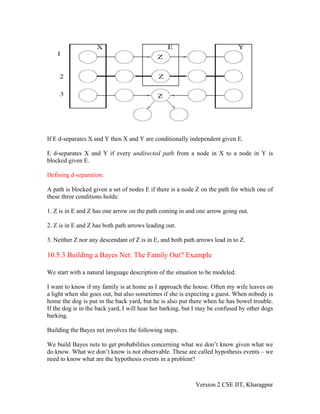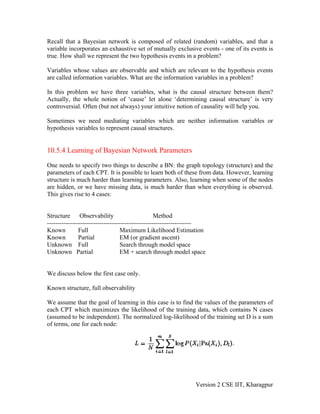Publicité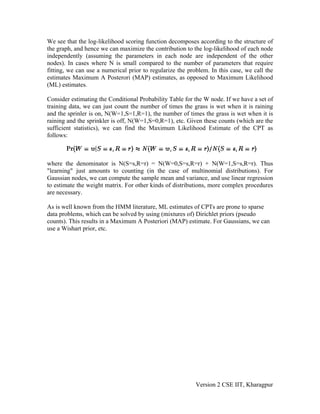Prochain SlideShare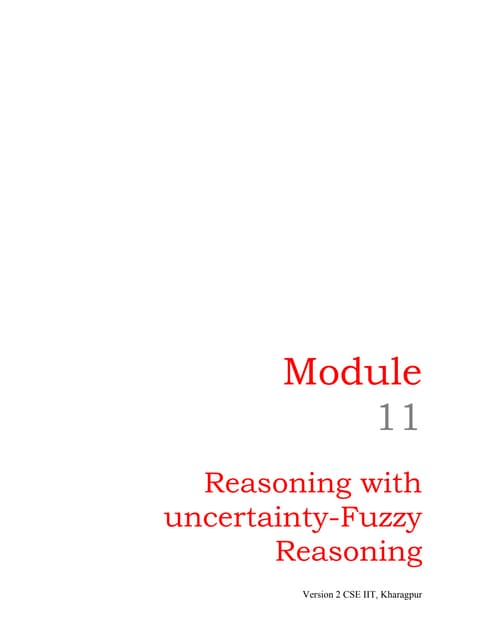Lesson 31
Chargement dans ... 3
1 sur 10
Publicité

### Lesson 28

1. Module 10 Reasoning with Uncertainty - Probabilistic reasoning Version 2 CSE IIT, Kharagpur
2. Lesson 28 Bayes Networks Version 2 CSE IIT, Kharagpur
3. 10.5 Bayesian Networks 10.5.1 Representation and Syntax Bayes nets (BN) (also referred to as Probabilistic Graphical Models and Bayesian Belief Networks) are directed acyclic graphs (DAGs) where each node represents a random variable. The intuitive meaning of an arrow from a parent to a child is that the parent directly influences the child. These influences are quantified by conditional probabilities. BNs are graphical representations of joint distributions. The BN for the medical expert system mentioned previously represents a joint distribution over 8 binary random variables {A,T,E,L,S,B,D,X}. Conditional Probability Tables Each node in a Bayesian net has an associated conditional probability table or CPT. (Assume all random variables have only a finite number of possible values). This gives the probability values for the random variable at the node conditional on values for its parents. Here is a part of one of the CPTs from the medical expert system network. If a node has no parents, then the CPT reduces to a table giving the marginal distribution on that random variable. Version 2 CSE IIT, Kharagpur
4. Consider another example, in which all nodes are binary, i.e., have two possible values, which we will denote by T (true) and F (false). We see that the event "grass is wet" (W=true) has two possible causes: either the water sprinker is on (S=true) or it is raining (R=true). The strength of this relationship is shown in the table. For example, we see that Pr(W=true | S=true, R=false) = 0.9 (second row), and hence, Pr(W=false | S=true, R=false) = 1 - 0.9 = 0.1, since each row must sum to one. Since the C node has no parents, its CPT specifies the prior probability that it is cloudy (in this case, 0.5). (Think of C as representing the season: if it is a cloudy season, it is less likely that the sprinkler is on and more likely that the rain is on.) 10.5.2 Semantics of Bayesian Networks The simplest conditional independence relationship encoded in a Bayesian network can be stated as follows: a node is independent of its ancestors given its parents, where the ancestor/parent relationship is with respect to some fixed topological ordering of the nodes. In the sprinkler example above, by the chain rule of probability, the joint probability of all the nodes in the graph above is Version 2 CSE IIT, Kharagpur
5. P(C, S, R, W) = P(C) * P(S|C) * P(R|C,S) * P(W|C,S,R) By using conditional independence relationships, we can rewrite this as P(C, S, R, W) = P(C) * P(S|C) * P(R|C) * P(W|S,R) where we were allowed to simplify the third term because R is independent of S given its parent C, and the last term because W is independent of C given its parents S and R. We can see that the conditional independence relationships allow us to represent the joint more compactly. Here the savings are minimal, but in general, if we had n binary nodes, the full joint would require O(2^n) space to represent, but the factored form would require O(n 2^k) space to represent, where k is the maximum fan-in of a node. And fewer parameters makes learning easier. The intuitive meaning of an arrow from a parent to a child is that the parent directly influences the child. The direction of this influence is often taken to represent casual influence. The conditional probabilities give the strength of causal influence. A 0 or 1 in a CPT represents a deterministic influence. 10.5.2.1 Decomposing Joint Distributions A joint distribution can always be broken down into a product of conditional probabilities using repeated applications of the product rule. We can order the variables however we like: 10.5.2.2 Conditional Independence in Bayes Net A Bayes net represents the assumption that each node is conditionally independent of all its non-descendants given its parents. So for example, Version 2 CSE IIT, Kharagpur
6. Note that, a node is NOT independent of its descendants given its parents. Generally, 10.5.2.3 Variable ordering in Bayes Net The conditional independence assumptions expressed by a Bayes net allow a compact representation of the joint distribution. First note that the Bayes net imposes a partial order on nodes: X <= Y iff X is a descendant of Y. We can always break down the joint so that the conditional probability factor for a node only has non-descendants in the condition. Version 2 CSE IIT, Kharagpur
7. 10.5.2.4 The Joint Distribution as a Product of CPTs Because each node is conditionally independent of all its nondescendants given its parents, and because we can write the joint appropriately we have: So the CPTs determine the full joint distribution. In short, Bayesian Networks allow a compact representation of the probability distributions. An unstructured table representation of the “medical expert system” joint would require 28 − 1 = 255 numbers. With the structure imposed by the conditional independence assumptions this reduces to 18 numbers. Structure also allows efficient inference — of which more later. 10.5.2.5 Conditional Independence and d-separation in a Bayesian Network We can have conditional independence relations between sets of random variables. In the Medical Expert System Bayesian net, {X, D} is independent of {A, T, L, S} given {E,B} which means: P(X, D | E, B) = P(X,D | E, B, A, T, L, S) equivalently . . . P(X, D, A, T, L, S | E, B) = P(A, T, L, S | E, B)P(X, D | E, B) We need a way of checking for these conditional independence relations Conditional independence can be checked uing the d-separation property of the Bayes net directed acyclic graph. d-separation is short for direction-dependent separation. Version 2 CSE IIT, Kharagpur
8. If E d-separates X and Y then X and Y are conditionally independent given E. E d-separates X and Y if every undirected path from a node in X to a node in Y is blocked given E. Defining d-separation: A path is blocked given a set of nodes E if there is a node Z on the path for which one of these three conditions holds: 1. Z is in E and Z has one arrow on the path coming in and one arrow going out. 2. Z is in E and Z has both path arrows leading out. 3. Neither Z nor any descendant of Z is in E, and both path arrows lead in to Z. 10.5.3 Building a Bayes Net: The Family Out? Example We start with a natural language description of the situation to be modeled: I want to know if my family is at home as I approach the house. Often my wife leaves on a light when she goes out, but also sometimes if she is expecting a guest. When nobody is home the dog is put in the back yard, but he is also put there when he has bowel trouble. If the dog is in the back yard, I will hear her barking, but I may be confused by other dogs barking. Building the Bayes net involves the following steps. We build Bayes nets to get probabilities concerning what we don’t know given what we do know. What we don’t know is not observable. These are called hypothesis events – we need to know what are the hypothesis events in a problem? Version 2 CSE IIT, Kharagpur
9. Recall that a Bayesian network is composed of related (random) variables, and that a variable incorporates an exhaustive set of mutually exclusive events - one of its events is true. How shall we represent the two hypothesis events in a problem? Variables whose values are observable and which are relevant to the hypothesis events are called information variables. What are the information variables in a problem? In this problem we have three variables, what is the causal structure between them? Actually, the whole notion of ‘cause’ let alone ‘determining causal structure’ is very controversial. Often (but not always) your intuitive notion of causality will help you. Sometimes we need mediating variables which are neither information variables or hypothesis variables to represent causal structures. 10.5.4 Learning of Bayesian Network Parameters One needs to specify two things to describe a BN: the graph topology (structure) and the parameters of each CPT. It is possible to learn both of these from data. However, learning structure is much harder than learning parameters. Also, learning when some of the nodes are hidden, or we have missing data, is much harder than when everything is observed. This gives rise to 4 cases: Structure Observability Method --------------------------------------------------------------------- Known Full Maximum Likelihood Estimation Known Partial EM (or gradient ascent) Unknown Full Search through model space Unknown Partial EM + search through model space We discuss below the first case only. Known structure, full observability We assume that the goal of learning in this case is to find the values of the parameters of each CPT which maximizes the likelihood of the training data, which contains N cases (assumed to be independent). The normalized log-likelihood of the training set D is a sum of terms, one for each node: Version 2 CSE IIT, Kharagpur
10. We see that the log-likelihood scoring function decomposes according to the structure of the graph, and hence we can maximize the contribution to the log-likelihood of each node independently (assuming the parameters in each node are independent of the other nodes). In cases where N is small compared to the number of parameters that require fitting, we can use a numerical prior to regularize the problem. In this case, we call the estimates Maximum A Posterori (MAP) estimates, as opposed to Maximum Likelihood (ML) estimates. Consider estimating the Conditional Probability Table for the W node. If we have a set of training data, we can just count the number of times the grass is wet when it is raining and the sprinler is on, N(W=1,S=1,R=1), the number of times the grass is wet when it is raining and the sprinkler is off, N(W=1,S=0,R=1), etc. Given these counts (which are the sufficient statistics), we can find the Maximum Likelihood Estimate of the CPT as follows: where the denominator is N(S=s,R=r) = N(W=0,S=s,R=r) + N(W=1,S=s,R=r). Thus "learning" just amounts to counting (in the case of multinomial distributions). For Gaussian nodes, we can compute the sample mean and variance, and use linear regression to estimate the weight matrix. For other kinds of distributions, more complex procedures are necessary. As is well known from the HMM literature, ML estimates of CPTs are prone to sparse data problems, which can be solved by using (mixtures of) Dirichlet priors (pseudo counts). This results in a Maximum A Posteriori (MAP) estimate. For Gaussians, we can use a Wishart prior, etc. Version 2 CSE IIT, Kharagpur
Publicité